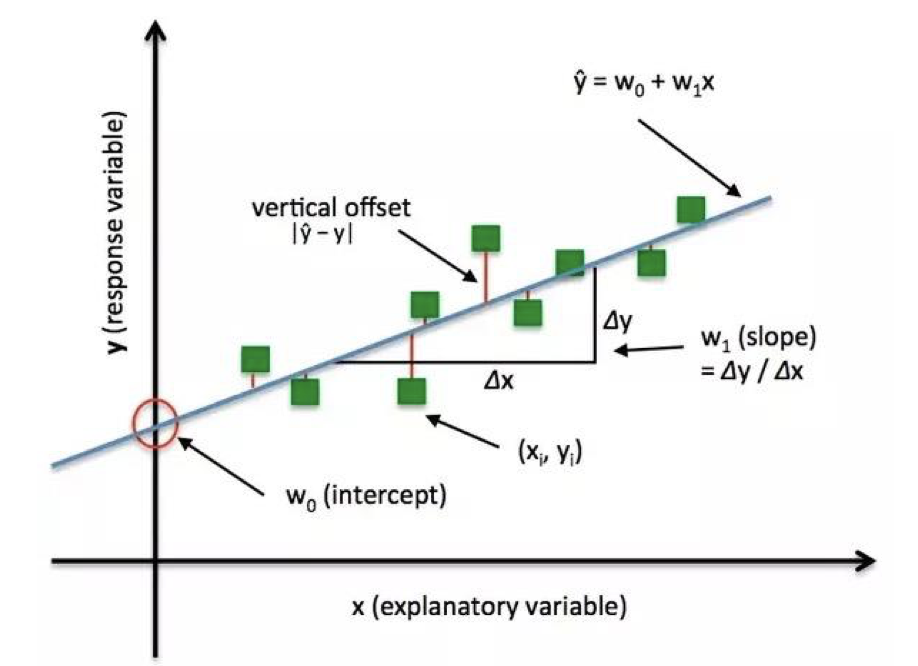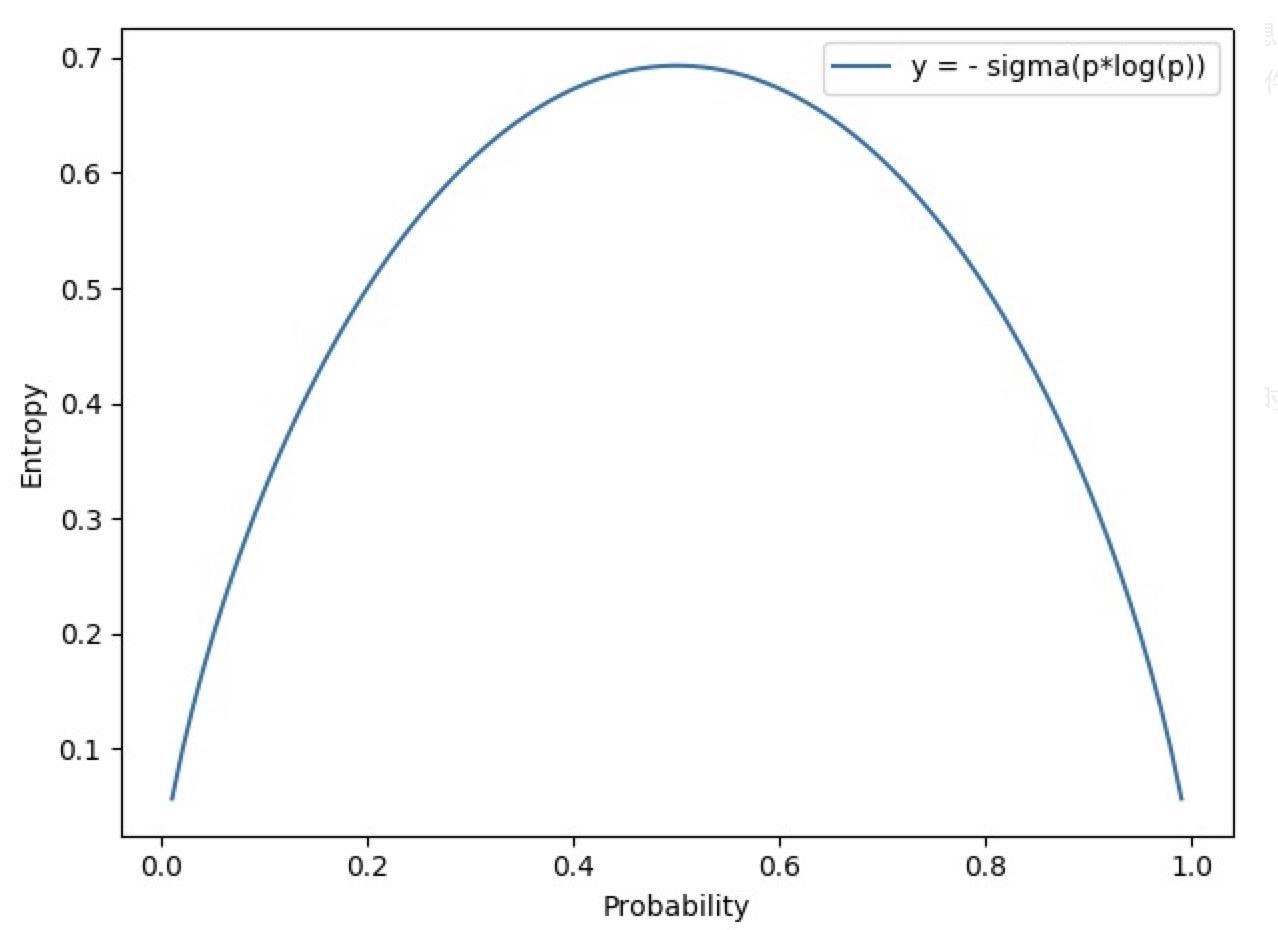# PyTorch 15：损失函数 (一)

## PyTorch 学习笔记

Posted by YEY on December 18, 2020

# Lecture 15 损失函数 (一)

## 1. 损失函数的概念• 损失函数 (Loss Function)：计算单个样本的差异。

$\mathrm{Loss} = f(\hat y, y)$
• 代价函数 (Cost Function)：计算整个训练集 $\mathrm{Loss}$ 的平均值。

$\mathrm{Cost} = \dfrac{1}{n}\sum_{i=1}^{n} f(\hat y_i, y_i)$
• 目标函数 (Objective Function)：最终需要优化的目标，通常包含代价函数和正则项。

$\mathrm{Obj} = \mathrm{Cost} + \mathrm{Regularization}$

1
2
3
4
5
6
7
class _Loss(Module):
def __init__(self, size_average=None, reduce=None, reduction='mean'):
super(_Loss, self).__init__()
if size_average is not None or reduce is not None:
self.reduction = _Reduction.legacy_get_string(size_average, reduce)
else:
self.reduction = reduction


## 2. 交叉熵损失函数

• 自信息 (Self-information)：用于衡量单个事件的不确定性。

$I(X) = -\log [P(X)]$

其中，$P(X)$ 为事件 $X$ 的概率。

• 熵 (Entropy)：自信息的期望，用于描述整个概率分布的不确定性。事件的不确定性越高，它的熵就越大。

$H(P) = \mathrm{E}_{X\sim P}[I(X)] = \sum_{i=1}^{n}P(x_i)\log P(x_i)$• 相对熵 (Relative Entropy)：又称 KL 散度 (Kullback-Leibler Divergence, KLD)，用于衡量两个概率分布之间的差异 (或者说距离)。注意，虽然 KL 散度可以衡量两个分布之间的距离，但它本身并不是一个距离函数，因为距离函数具有对称性，即 $P$ 到 $Q$ 的距离必须等于 $Q$ 到 $P$ 的距离，而相对熵不具备这种对称性。

$D_{\mathrm{KL}}(P, Q) = \mathrm{E}_{X \sim P}\left[\log \dfrac{P(X)}{Q(X)}\right]$

其中，$P$ 是数据的真实分布，$Q$ 是模型拟合的分布，二者定义在相同的概率空间上。我们需要用拟合分布 $Q$ 去逼近真实分布 $P$，所以相对熵不具备对称性。

• 交叉熵 (Cross Entropy)：用于衡量两个分布之间的相似度。

$H(P,Q)= -\sum_{i=1}^{n}P(x_i)\log Q(x_i)$

\begin{aligned} D_{\mathrm{KL}}(P, Q) &= \mathrm{E}_{X \sim P}\left[\log \dfrac{P(X)}{Q(X)}\right] \\[2ex] &= \mathrm{E}_{X \sim P} [\log P(X) - \log Q(X) ] \\[2ex] &= \sum_{i=1}^{n} P(x_i) [\log P(x_i) - \log Q(x_i) ] \\[2ex] &= \sum_{i=1}^{n} P(x_i) \log P(x_i) - \sum_{i=1}^{n} P(x_i) \log Q(x_i) \\[2ex] &= H(P, Q) - H(P) \end{aligned}

$H(P, Q) = H(P) + D_{\mathrm{KL}}(P, Q)$

#### nn.CrossEntropyLoss

1
2
3
4
5
6
7
nn.CrossEntropyLoss(
weight=None,
size_average=None,
ignore_index=-100,
reduce=None,
reduction='mean'
)


• weight：各类别的 loss 设置权值。
• ignore_index：忽略某个类别，不计算其 loss。
• reduction：计算模式，可为 none/sum/mean
• none：逐个元素计算。
• sum：所有元素求和，返回标量。
• mean：加权平均，返回标量。

PyTorch 中 nn.CrossEntropyLoss 的交叉熵计算公式

• 没有针对各类别 loss 设置权值的情况：

$\mathrm{loss}(x, class) = -\log \left(\dfrac{\exp(x[class])}{\sum_j \exp(x[j])} \right) = -x[class] + \log \left(\sum_j \exp(x[j])\right)$
• 对各类别 loss 设置权值的情况：

$\mathrm{loss}(x, class) = \mathrm{weight}[class] \left(-x[class] + \log \left(\sum_j \exp(x[j])\right)\right)$

$H(P,Q)= -\sum_{i=1}^{n}P(x_i)\log Q(x_i)$

$H(P,Q)= -\log Q(x_i)$

1
2
3
4
5
6
7
8
9
10
11
12
13
14
15
16
17
18
19
20
21
import torch
import torch.nn as nn
import numpy as np

# fake data
inputs = torch.tensor([[1, 2], [1, 3], [1, 3]], dtype=torch.float)
target = torch.tensor([0, 1, 1], dtype=torch.long)  # 注意 label 在这里必须设置为长整型

# ------------------------ CrossEntropy loss: reduction ----------------------
# def loss function
loss_f_none = nn.CrossEntropyLoss(weight=None, reduction='none')
loss_f_sum = nn.CrossEntropyLoss(weight=None, reduction='sum')
loss_f_mean = nn.CrossEntropyLoss(weight=None, reduction='mean')

# forward
loss_none = loss_f_none(inputs, target)
loss_sum = loss_f_sum(inputs, target)
loss_mean = loss_f_mean(inputs, target)

# view
print("Cross Entropy Loss:\n ", loss_none, loss_sum, loss_mean)


1
2
Cross Entropy Loss:
tensor([1.3133, 0.1269, 0.1269]) tensor(1.5671) tensor(0.5224)


$\mathrm{loss}(x, class) = -x[class] + \log \left(\sum_j \exp(x[j])\right)$
1
2
3
4
5
6
7
8
9
10
11
12
13
14
15
16
idx = 0

input_1 = inputs.detach().numpy()[idx]      # [1, 2]
target_1 = target.numpy()[idx]              # 

# 第一项
x_class = input_1[target_1]

# 第二项
sigma_exp_x = np.sum(list(map(np.exp, input_1)))
log_sigma_exp_x = np.log(sigma_exp_x)

# 输出loss
loss_1 = -x_class + log_sigma_exp_x

print("第一个样本的 loss 为: ", loss_1)


1



1
2
3
4
5
6
7
8
9
10
11
12
13
14
15
16
17
18
# def loss function
# 向量长度应该与类别数量一致，如果 reduction 参数为 'mean'，那么我们不需要关注
# weight 的尺度，只需要关注各类别的 weight 比例即可。
weights = torch.tensor([1, 2], dtype=torch.float)
# weights = torch.tensor([0.7, 0.3], dtype=torch.float)

loss_f_none_w = nn.CrossEntropyLoss(weight=weights, reduction='none')
loss_f_sum = nn.CrossEntropyLoss(weight=weights, reduction='sum')
loss_f_mean = nn.CrossEntropyLoss(weight=weights, reduction='mean')

# forward
loss_none_w = loss_f_none_w(inputs, target)
loss_sum = loss_f_sum(inputs, target)
loss_mean = loss_f_mean(inputs, target)

# view
print("\nweights: ", weights)
print(loss_none_w, loss_sum, loss_mean)


1
2
weights:  tensor([1., 2.])
tensor([1.3133, 0.2539, 0.2539]) tensor(1.8210) tensor(0.3642)


1
2
3
4
5
6
7
8
9
10
11
12
weights = torch.tensor([1, 2], dtype=torch.float)
weights_all = np.sum(list(map(lambda x: weights.numpy()[x], target.numpy())))

mean = 0
loss_sep = loss_none.detach().numpy()

for i in range(target.shape):
x_class = target.numpy()[i]
tmp = loss_sep[i] * (weights.numpy()[x_class] / weights_all)
mean += tmp

print(mean)


1
0.3641947731375694


## 3. NLL/BCE/BCEWithLogits Loss

#### nn.NLLLoss

1
2
3
4
5
6
7
nn.NLLLoss(
weight=None,
size_average=None,
ignore_index=-100,
reduce=None,
reduction='mean'
)


• weight：各类别的 loss 设置权值。
• ignore_index：忽略某个类别。
• reduction：计算模式，可为 none/sum/mean
• none：逐个元素计算。
• sum：所有元素求和，返回标量。
• mean：加权平均，返回标量。

$\ell(x,y) = L = \{l_1,\dots,l_N\}^{\mathrm T}\;,\qquad l_n = -w_{y_n}x_{n,y_n}$

1
2
3
4
5
6
7
8
9
10
11
12
13
14
15
16
17
18
19
20
# fake data, 这里我们使用的还是之前的数据，注意 label 在这里必须设置为 long
inputs = torch.tensor([[1, 2], [1, 3], [1, 3]], dtype=torch.float)
target = torch.tensor([0, 1, 1], dtype=torch.long)

# weights
weights = torch.tensor([1, 1], dtype=torch.float)

# NLL loss
loss_f_none_w = nn.NLLLoss(weight=weights, reduction='none')
loss_f_sum = nn.NLLLoss(weight=weights, reduction='sum')
loss_f_mean = nn.NLLLoss(weight=weights, reduction='mean')

# forward
loss_none_w = loss_f_none_w(inputs, target)
loss_sum = loss_f_sum(inputs, target)
loss_mean = loss_f_mean(inputs, target)

# view
print("\nweights: ", weights)
print("NLL Loss", loss_none_w, loss_sum, loss_mean)


1
2
weights:  tensor([1., 1.])
NLL Loss tensor([-1., -3., -3.]) tensor(-7.) tensor(-2.3333)


#### nn.BCELoss

1
2
3
4
5
6
nn.BCELoss(
weight=None,
size_average=None,
reduce=None,
reduction='mean'
)


• weight：各类别的 loss 设置权值。
• ignore_index：忽略某个类别。
• reduction：计算模式，可为 none/sum/mean
• none：逐个元素计算。
• sum：所有元素求和，返回标量。
• mean：加权平均，返回标量。

$l_n = -w_n [y_n \cdot \log x_n + (1- y_n) \cdot \log(1-x_n)]$

1
2
3
4
5
6
7
8
9
10
11
12
13
14
15
16
17
18
19
20
21
22
23
24
25
# fake data, 这里我们设置 4 个样本，注意 label 在这里必须设置为 float
inputs = torch.tensor([[1, 2], [2, 2], [3, 4], [4, 5]], dtype=torch.float)
target = torch.tensor([[1, 0], [1, 0], [0, 1], [0, 1]], dtype=torch.float)

target_bce = target

# itarget
inputs = torch.sigmoid(inputs)  # 利用 Sigmoid 函数将输入值压缩到 [0,1]

# weights
weights = torch.tensor([1, 1], dtype=torch.float)

# BCE loss
loss_f_none_w = nn.BCELoss(weight=weights, reduction='none')
loss_f_sum = nn.BCELoss(weight=weights, reduction='sum')
loss_f_mean = nn.BCELoss(weight=weights, reduction='mean')

# forward
loss_none_w = loss_f_none_w(inputs, target_bce)
loss_sum = loss_f_sum(inputs, target_bce)
loss_mean = loss_f_mean(inputs, target_bce)

# view
print("\nweights: ", weights)
print("BCE Loss", loss_none_w, loss_sum, loss_mean)


1
2
3
4
5
weights:  tensor([1., 1.])
BCE Loss tensor([[0.3133, 2.1269],
[0.1269, 2.1269],
[3.0486, 0.0181],
[4.0181, 0.0067]]) tensor(11.7856) tensor(1.4732)


1
2
3
4
5
6
7
8
9
10
11
12
idx = 0

x_i = inputs.detach().numpy()[idx, idx]  # 获取第一个神经元的输出值
y_i = target.numpy()[idx, idx]  # 获取第一个神经元的标签

# loss
# l_i = -[ y_i * np.log(x_i) + (1-y_i) * np.log(1-y_i) ]      # np.log(0) = nan
l_i = -y_i * np.log(x_i) if y_i else -(1-y_i) * np.log(1-x_i)

# 输出loss
print("BCE inputs: ", inputs)
print("第一个 loss 为: ", l_i)


1
2
3
4
5
BCE inputs:  tensor([[0.7311, 0.8808],
[0.8808, 0.8808],
[0.9526, 0.9820],
[0.9820, 0.9933]])



#### nn.BCEWithLogitsLoss

1
2
3
4
5
6
7
nn.BCEWithLogitsLoss(
weight=None,
size_average=None,
reduce=None,
reduction='mean',
pos_weight=None
)


• pos_weight：正样本的权值，用于平衡正负样本。
• 例如：正样本有 100 个，负样本有 300 个，正负样本比例为 $1:3$。因此我们可以将该项设为 $3$，这样即等价于正负样本各 300 个。
• weight：各类别的 loss 设置权值。
• ignore_index：忽略某个类别。
• reduction：计算模式，可为 none/sum/mean
• none：逐个元素计算。
• sum：所有元素求和，返回标量。
• mean：加权平均，返回标量。

$l_n = -w_n[y_n \cdot \log \sigma(x_n) + (1-y_n)\cdot \log (1-\sigma (x_n))]$

1
2
3
4
5
6
7
8
9
10
11
12
13
14
15
16
17
18
19
20
21
inputs = torch.tensor([[1, 2], [2, 2], [3, 4], [4, 5]], dtype=torch.float)
target = torch.tensor([[1, 0], [1, 0], [0, 1], [0, 1]], dtype=torch.float)

target_bce = target

# inputs = torch.sigmoid(inputs)  # 这里增加 sigmoid 会使得计算不准确，因为相当于加了两层 sigmoid

weights = torch.tensor([1, 1], dtype=torch.float)

loss_f_none_w = nn.BCEWithLogitsLoss(weight=weights, reduction='none')
loss_f_sum = nn.BCEWithLogitsLoss(weight=weights, reduction='sum')
loss_f_mean = nn.BCEWithLogitsLoss(weight=weights, reduction='mean')

# forward
loss_none_w = loss_f_none_w(inputs, target_bce)
loss_sum = loss_f_sum(inputs, target_bce)
loss_mean = loss_f_mean(inputs, target_bce)

# view
print("\nweights: ", weights)
print(loss_none_w, loss_sum, loss_mean)


1
2
3
4
5
weights:  tensor([1., 1.])
tensor([[0.3133, 2.1269],
[0.1269, 2.1269],
[3.0486, 0.0181],
[4.0181, 0.0067]]) tensor(11.7856) tensor(1.4732)


1
2
3
4
5
6
7
8
9
10
11
12
13
14
15
16
17
18
19
20
inputs = torch.tensor([[1, 2], [2, 2], [3, 4], [4, 5]], dtype=torch.float)
target = torch.tensor([[1, 0], [1, 0], [0, 1], [0, 1]], dtype=torch.float)

target_bce = target

weights = torch.tensor(, dtype=torch.float)
pos_w = torch.tensor(, dtype=torch.float)  # 将 pos_weight 设为 1

loss_f_none_w = nn.BCEWithLogitsLoss(weight=weights, reduction='none', pos_weight=pos_w)
loss_f_sum = nn.BCEWithLogitsLoss(weight=weights, reduction='sum', pos_weight=pos_w)
loss_f_mean = nn.BCEWithLogitsLoss(weight=weights, reduction='mean', pos_weight=pos_w)

# forward
loss_none_w = loss_f_none_w(inputs, target_bce)
loss_sum = loss_f_sum(inputs, target_bce)
loss_mean = loss_f_mean(inputs, target_bce)

# view
print("\npos_weights: ", pos_w)
print(loss_none_w, loss_sum, loss_mean)


1
2
3
4
5
pos_weights:  tensor([1.])
tensor([[0.3133, 2.1269],
[0.1269, 2.1269],
[3.0486, 0.0181],
[4.0181, 0.0067]]) tensor(11.7856) tensor(1.4732)


1
pos_w = torch.tensor(, dtype=torch.float)  # 将 pos_weight 设为 3


1
2
3
4
5
pos_weights:  tensor([3.])
tensor([[0.9398, 2.1269],
[0.3808, 2.1269],
[3.0486, 0.0544],
[4.0181, 0.0201]]) tensor(12.7158) tensor(1.5895)


## 4. 总结本作品采用知识共享署名-非商业性使用-相同方式共享 4.0 国际许可协议进行许可。 欢迎转载，并请注明来自：YEY 的博客 同时保持文章内容的完整和以上声明信息！